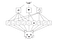# Deep Learning in 5 minutes Part 1: Convolutional Neural Networks

In this series of posts, I have only one objective; present core concepts of deep learning in five minutes. This is the first post of the series dedicated to convolutional neural networks.

Convolutional neural networks, ConvNets or CNN allow deep networks to learn functions on structured spatial data such as images, video, and text. Mathematically, convolutional networks provide tools for exploiting the local structure of data effectively. 

## Why are ConvNets useful?

They have became popular due to this reasons:

• No feature extraction because features are learned directly in CNN.

There are four main operations in a ConvNet :

1. Convolution

## Convolution

In its most general form, convolution is an operation on two functions of a real-valued argument.

A convolutional kernel is just a matrix of weights, much like the weights associated with a fully connected layer. A convolutional kernel is applied to inputs. The kernel weights are multiplied elementwise with the corresponding numbers in the local receptive field.

The local receptive field (input feature) is a local region of the input volume that has the same size as the filter. The filter, kernel, or feature detector is a small matrix used for features detection and the convolved feature, activation map or feature map is the output volume formed by sliding the filter over the image and computing the dot product . Generally, the input is a multidimensional array of parameters, in practice, we can implement the infinite summation as a summation over a finite number of array elements .

## Non Linearity (ReLU)

ReLU means Rectified Linear Unit for a non-linear operation. The output is ƒ(x) = max(0,x).

## Pooling or Sub-sampling

Pooling layers are useful for reducing the dimensionality of input data in a structured way. They take a local receptive field and replace the nonlinear activation function at each portion of the field with the max (or min or average) function. The most common is the max pooling. [1, 6]

## Fully Conected Layer (Classification layer)

Fully connected layers connect every neuron in one layer to every neuron in another layer. The last fully-connected layer uses a softmax activation function for classifying the generated features of the input image into various classes based on the training dataset.

And strides, what about the strides?

Stride is the number of pixels shifts over the input matrix. When the stride is 1 then we move the filters to 1 pixel at a time. When the stride is 2 then we move the filters to 2 pixels at a time and so on. The below figure shows convolution would work with a stride of 2. 

## Important Notes

CNN has two interesting properties:

1. The patterns learned are translation invariant. After recognizing a pattern in a picture, it can recognize anywhere. They need fewer training images to learn representations.Spatial hierarchy is learned by CNN. Source: Deep Learning with Python 

## Applications

1. Object Detection and Localization: is the task of detecting the objects (or identities) present in a photograph, then draws a “bounding box” around the object. Useful for detecting pedestrians in images for self-driving cars.
1. Image segmentation: related to object detection but a little bit harder, because it requires the precise understanding of the boundaries between objects and images. Previously, was done with graphical models.
1. Graph Convolutions: in chemistry molecules can be modeled as undirected graphs where atoms form nodes and chemical bonds form edges. 

# Resources

1. Ramsundar, B; Zadeth, R. Tensorflow for Deep Learning. 2018

2. Convolutional Neural Network. 3 things you need to know http://bit.ly/2TL3dEB

3. An Intuitive Explanation of Convolutional Neural Networks http://bit.ly/2TJ0PhQ

4. Godfellow, I; Bengio, Y; Courville, A. Deep Learning. 2015

5. Chollet, F. Deep Learning with Python. 2017

6. Understanding of Convolutional Neural Network (CNN) — Deep Learning http://bit.ly/2AolUp3

7. Udacity videos playlist on Youtube http://bit.ly/2AoZaoy

Written by